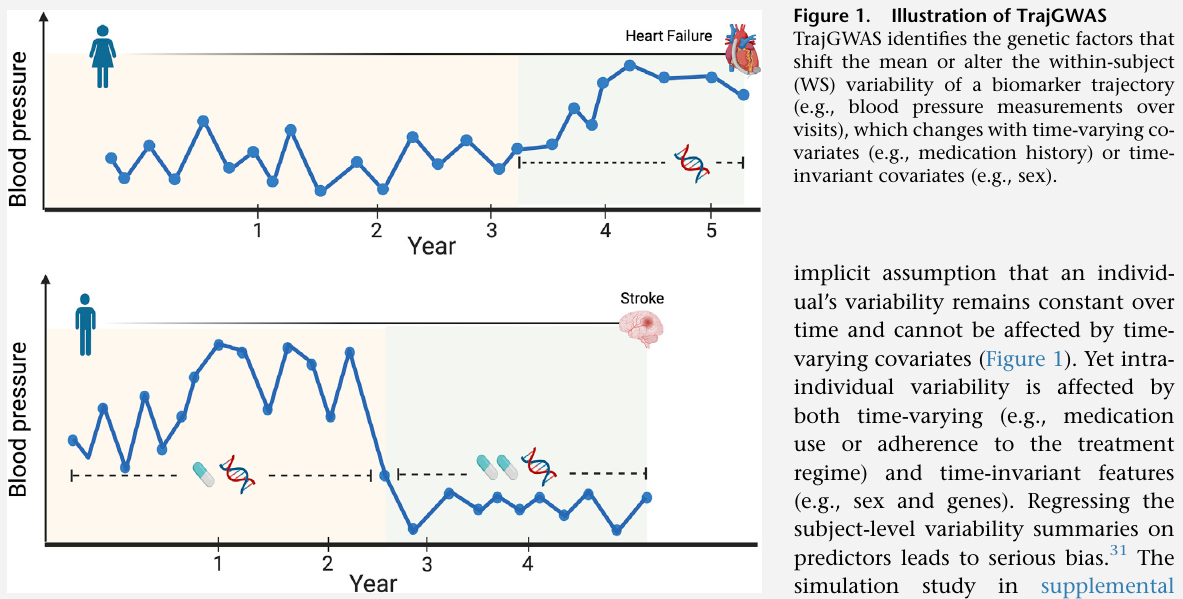# GWAS of Longitudinal Trajectories at Biobank Scale

##### Posted on Jun 11, 2023 (Update: Jun 28, 2023)
Tags: GWAS, Longitudinal, BiobankRecent research reveals that not only the mean level of biomarker trajectories but also their fluctuations, or within-subject (WS) variability, are risk factors for many diseases.

It is crucial to identify the genetic factors that shift the mean or alter the WS variability of a biomarker trajectory.

Currently, no efficient tools for GWAS of biomarker trajectories at the biobank scale, even for just mean effects.

The paper proposes TrajGWAS, a linear mixed effect model-based method for testing genetic effects that shift the mean or alter the WS variability of a biomarker trajectory.

Assume there are $m$ independent individuals, individual $i$ has $n_i$ longitudinal measurements of a biomarker, and $n = \sum_{i=1}^m n_i$ is the total number of observations.

Consider an LMM for modeling different sources of variation in a biomarker in the longitudinal setting,

$y_{ij} = \bfx_{ij}^T\bfbeta + g_i\beta_g + z_{ij}^T\gamma_i + \varepsilon_{ij}$

where

• $y_{ij}$: individual $i$’s measurement at occasion $j\in \{1,\ldots, n_i\}$
• $\bfx_{ij}$: $p\times 1$ vector of regressors with corresponding regression coefficients $\bfbeta$
• $g_i$: genotype dosage of individual $i$ with corresponding genetic mean effect $\beta_g$
• the WS variability is captured by the random terms $\varepsilon_{ij}$ with mean zero and inhomogeneous variance $\sigma^2_{\varepsilon_{ij}} = \exp(w_{ij}^T\tau + g_i\tau_g +\omega_i)$

Given a longitudinal biomarker of interest, the primary goal is to test

1. the mean effect of genotype, $H_0:\beta_g = 0$, i.e., whether a genotype shifts the mean of the biomarker trajectory
2. the WS variance effect of genotype, $H_0:\tau_g = 0$, i.e., whether a genotype changes the WS variation of the biomarker trajectory around its mean
3. the joint effect, $H_0: \beta_g = \tau_g = 0$, i.e., whether a genotype affects either the mean, or the WS variation, or both.

Published in categories Note